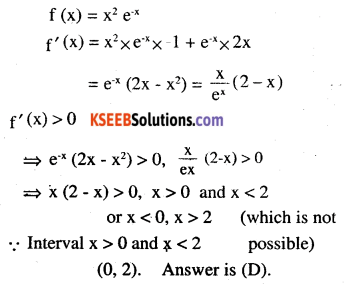# 2nd PUC Maths Question Bank Chapter 6 Application of Derivatives Ex 6.2

Students can Download Maths Chapter 6 Application of Derivatives Ex 6.2 Questions and Answers, Notes Pdf, 2nd PUC Maths Question Bank with Answers helps you to revise the complete Karnataka State Board Syllabus and score more marks in your examinations.

## Karnataka 2nd PUC Maths Question Bank Chapter 6 Application of Derivatives Ex 6.2

### 2nd PUC Maths Application of Derivatives NCERT Text Book Questions and Answers Ex 6.2

Question 1.
Find the intervals in which the function f given by f (x) = 3x + 17 is strictly increasing in R
f (x) = 3x + 17
f’ (x) = 3 > 0 , ∀ x ∈ R
hence f(x) is strictly increasing on R.

Question 2.
Show that the function given by f (x) = e2x is strightly increasing on R
f’ (x) = 2 e2x > 0 ∀ x ∈ R
hence f (x) is strictly increasing on R.Question 3.
Show that the function given by f(x) = sin x is
(a) strictly increasing in $$(0, \pi / 2)$$
(b) strictly decreasing in $$(\pi / 2, \pi)$$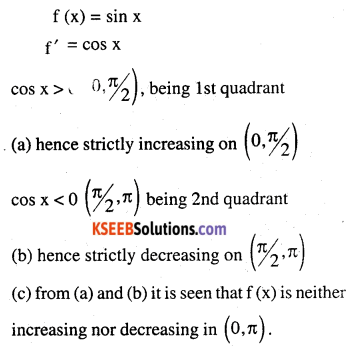Question 4.
Find the intervals in which the function f given by f (x) = 2x2 – 3x is
(a) strictly increasing
(b) strictly decreasingQuestion 5.
Find the intervals in which the function f given by f (x) = 2x2 – 3x2 – 36x +7 is
(a) strictly increasing
(b) strictly decreasing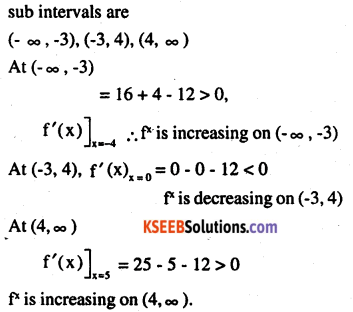Question 6.
Find the intervals in which the following functions are strictly increasing or decreasing:
(a) x2 + 2x – 5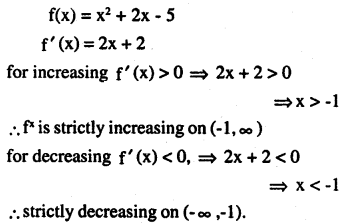(b) 10 – 6x – 2x2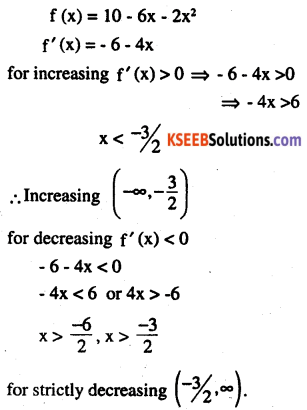(c) -2x3 – 9x2 – 12x + 1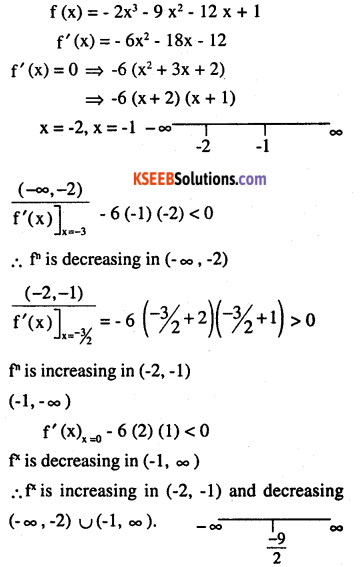(d) 6 – 9x – x2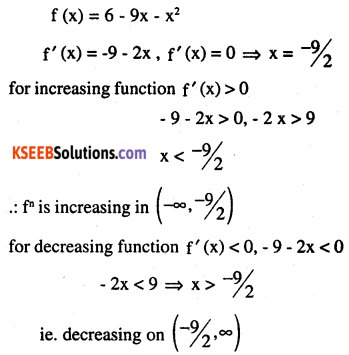(e) (x + 1)3 (x -3)3
f (x) = (x + 1)3 (x – 3)3
f’ (x) = (x + 1)3 x 3(x – 3)2 + (x – 3)3 x 3 (x + 1)2
= (x + 1)2 (x – 3)2 [3x + 3 + 3x – 9]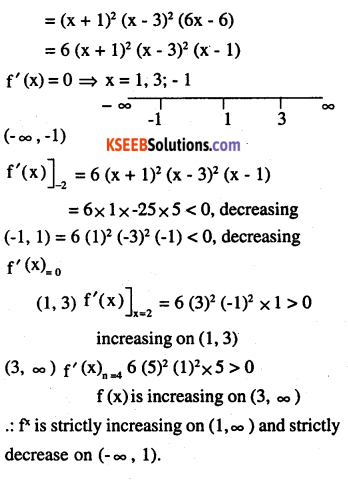Question 7.
Show that $$y=\log (1+x)-\frac{2 x}{2+x}, x>-1$$ an increasing function of x throughout its domain.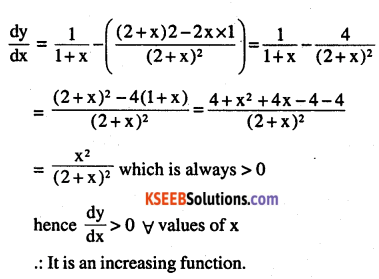Question 8.
Find the values of x for which y = [x(x – 2)]2 is an increasing function.Question 9.
Prove that $$y=\frac{4 \sin \theta}{(2+\cos \theta)}-\theta$$ is an a increasing function of θ in $$\left[0, \frac{\pi}{2}\right]$$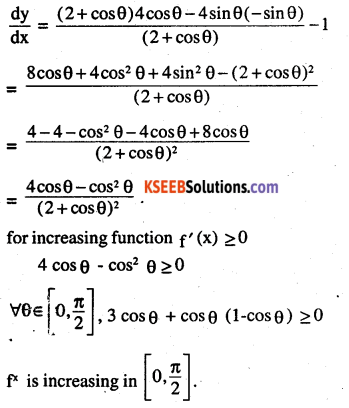Question 10.
Prove that the logarithmic function is strictly increasing on (0, ∞).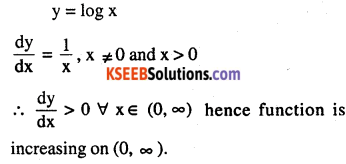Question 11.
Prove that the function f given by f (x) = x2 – x + 1 is neither strictly increasing nor strictly decreasing on (- 1, 1).Question 12.
Which of the following function are strictly decreases on $$(0, \pi / 2)$$
(a) cos x(b) f(x) = cos 2x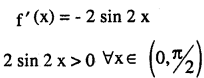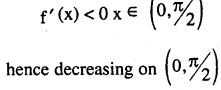(c) cos 3x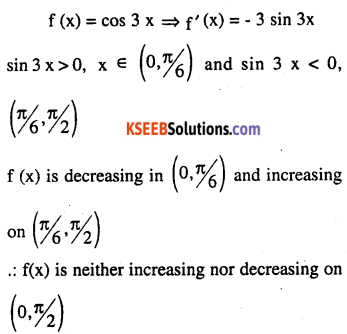(d) tan xQuestion 13.
On which of the following intervals is the function f given by f (x) = x100 + sin x – 1 strictly decreasing ?
(A) (0,1)
(B) $$\left(\frac{\pi}{2}, \pi\right)$$
(C) $$\left(0, \frac{\pi}{2}\right)$$
(D) None of theseQuestion 14.
Find the least value of a such that the function f given by f (x) = x2 + ax + 1 is strictly increasing on (1, 2).
f (x) = x2 + ax + 1
f’ (x) = 2x + a
f (x) is increasing if f’ (x) > 0
2x + a > 0 is x > – a/2
2x > -a – a < 2x ⇒ a > – 2x
since x ∈ (1,2) a > -2
The least value is -2.Question 15.
Let I be any interval disjoint from (-1, 1). Prove that the function f given by $$f(x)=x+\frac{1}{x}$$ is strictly increasing on I.Question 16.
Prove that the function f given by f (x) = log sin x is strictly increasing on
$$\left(0, \frac{\pi}{2}\right)$$ and strictly increasing on $$\left(0, \frac{\pi}{2}\right)$$and strictly decreasing on $$\left(\frac{\pi}{2}, \pi\right)$$Question 17.
Prove that the function f given by f (x) = log cos x is strictly decreasing on
$$\left(0, \frac{\pi}{2}\right)$$and strictly increasing on $$\left(\frac{\pi}{2}, \pi\right)$$Question 18.
Prove that the function given by f (x) = x3 – 3x2 + 3x – 100 is increasing in R.
f (x) = x3 – 3x2 + 3x
f'(x) = 3x2 – 6x + 3
= 3 (x – 2)2
f'(x)>0 ∀ x ∈ R
∴ function is continuous on RQuestion 19.
The interval in which y = x2 e-x is increasing is
(A) (- ∞ , ∞)
(B) ( – 2, 0)
(C) (2, ∞)
(D) (0,2).